Plotting¶

Introduction¶

Labeled data enables expressive computations. These same labels can also be used to easily create informative plots.

xarray’s plotting capabilities are centered around xarray.DataArray objects. To plot xarray.Dataset objects simply access the relevant DataArrays, ie dset['var1']. Here we focus mostly on arrays 2d or larger. If your data fits nicely into a pandas DataFrame then you’re better off using one of the more developed tools there.

xarray plotting functionality is a thin wrapper around the popular matplotlib library. Matplotlib syntax and function names were copied as much as possible, which makes for an easy transition between the two. Matplotlib must be installed before xarray can plot.

For more extensive plotting applications consider the following projects:

• Seaborn: “provides a high-level interface for drawing attractive statistical graphics.” Integrates well with pandas.
• Holoviews: “Composable, declarative data structures for building even complex visualizations easily.” Works for 2d datasets.
• Cartopy: Provides cartographic tools.

Imports¶

The following imports are necessary for all of the examples.

In : import numpy as np

In : import pandas as pd

In : import matplotlib.pyplot as plt

In : import xarray as xr

For these examples we’ll use the North American air temperature dataset.

In : airtemps = xr.tutorial.load_dataset('air_temperature')

In : airtemps
Out:
<xarray.Dataset>
Dimensions:  (lat: 25, lon: 53, time: 2920)
Coordinates:
* lat      (lat) float32 75.0 72.5 70.0 67.5 65.0 62.5 60.0 57.5 55.0 52.5 ...
* time     (time) datetime64[ns] 2013-01-01 2013-01-01T06:00:00 ...
* lon      (lon) float32 200.0 202.5 205.0 207.5 210.0 212.5 215.0 217.5 ...
Data variables:
air      (time, lat, lon) float64 241.2 242.5 243.5 244.0 244.1 243.9 ...
Attributes:
platform: Model
Conventions: COARDS
references: http://www.esrl.noaa.gov/psd/data/gridded/data.ncep.reanalysis.html
description: Data is from NMC initialized reanalysis
(4x/day).  These are the 0.9950 sigma level values.
title: 4x daily NMC reanalysis (1948)

# Convert to celsius
In : air = airtemps.air - 273.15

One Dimension¶

Simple Example¶

xarray uses the coordinate name to label the x axis.

In : air1d = air.isel(lat=10, lon=10)

In : air1d.plot()
Out: [<matplotlib.lines.Line2D at 0x7fb795613610>]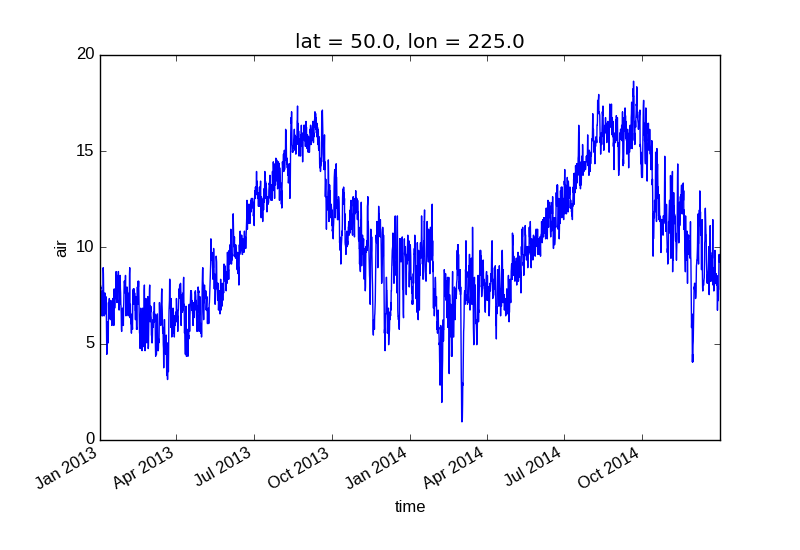Additional arguments are passed directly to the matplotlib function which does the work. For example, xarray.plot.line() calls matplotlib.pyplot.plot passing in the index and the array values as x and y, respectively. So to make a line plot with blue triangles a matplotlib format string can be used:

In : air1d[:200].plot.line('b-^')
Out: [<matplotlib.lines.Line2D at 0x7fb7956e8ad0>]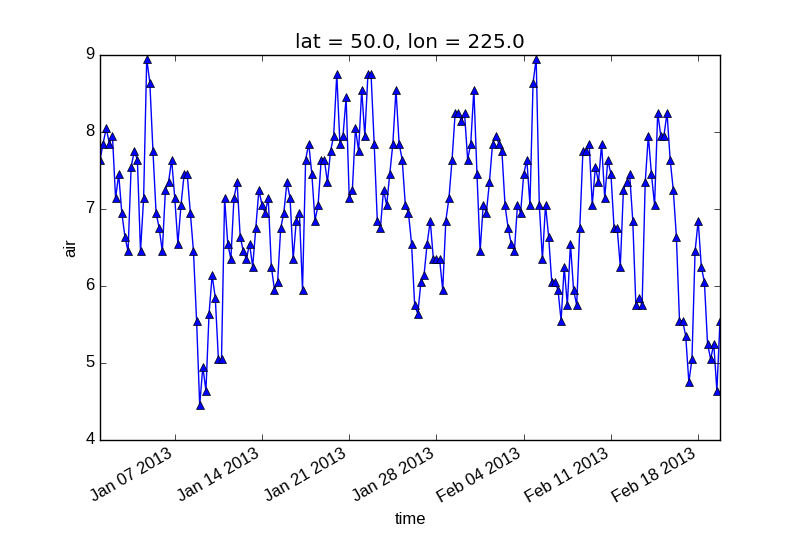Note

Not all xarray plotting methods support passing positional arguments to the wrapped matplotlib functions, but they do all support keyword arguments.

Keyword arguments work the same way, and are more explicit.

In : air1d[:200].plot.line(color='purple', marker='o')
Out: [<matplotlib.lines.Line2D at 0x7fb7950bf510>]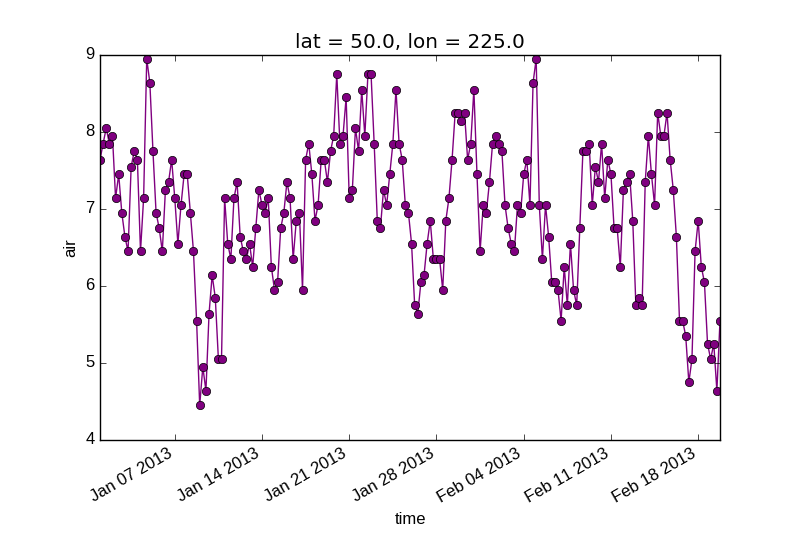Adding to Existing Axis¶

To add the plot to an existing axis pass in the axis as a keyword argument ax. This works for all xarray plotting methods. In this example axes is an array consisting of the left and right axes created by plt.subplots.

In : fig, axes = plt.subplots(ncols=2)

In : axes
Out:
array([<matplotlib.axes._subplots.AxesSubplot object at 0x7fb7951c2d50>,
<matplotlib.axes._subplots.AxesSubplot object at 0x7fb7955f7a90>], dtype=object)

In : air1d.plot(ax=axes)
Out: [<matplotlib.lines.Line2D at 0x7fb7954c2490>]

In : air1d.plot.hist(ax=axes)
Out:
(array([   9.,   38.,  255.,  584.,  542.,  489.,  368.,  258.,  327.,   50.]),
array([  0.95 ,   2.719,   4.488, ...,  15.102,  16.871,  18.64 ]),
<a list of 10 Patch objects>)

In : plt.tight_layout()

In : plt.show()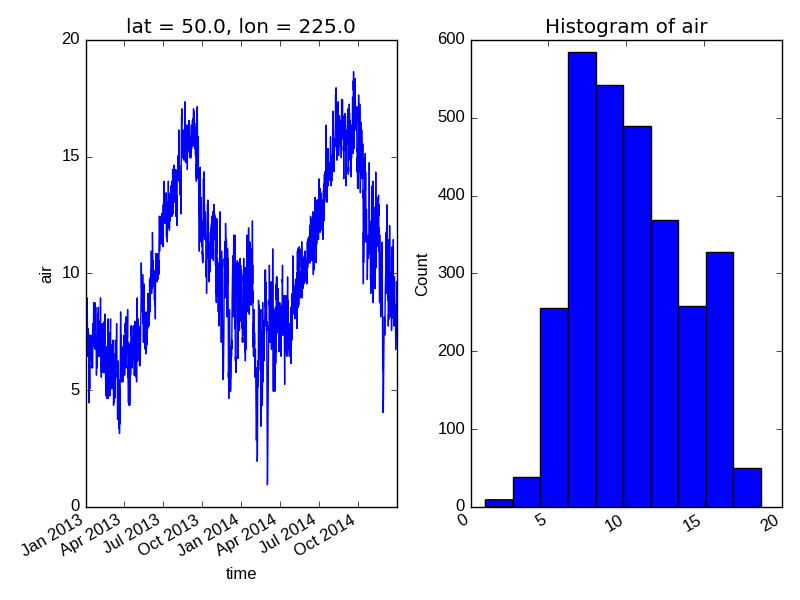On the right is a histogram created by xarray.plot.hist().

Two Dimensions¶

Simple Example¶

The default method xarray.DataArray.plot() sees that the data is 2 dimensional and calls xarray.plot.pcolormesh().

In : air2d = air.isel(time=500)

In : air2d.plot()
Out: <matplotlib.collections.QuadMesh at 0x7fb79df3d610>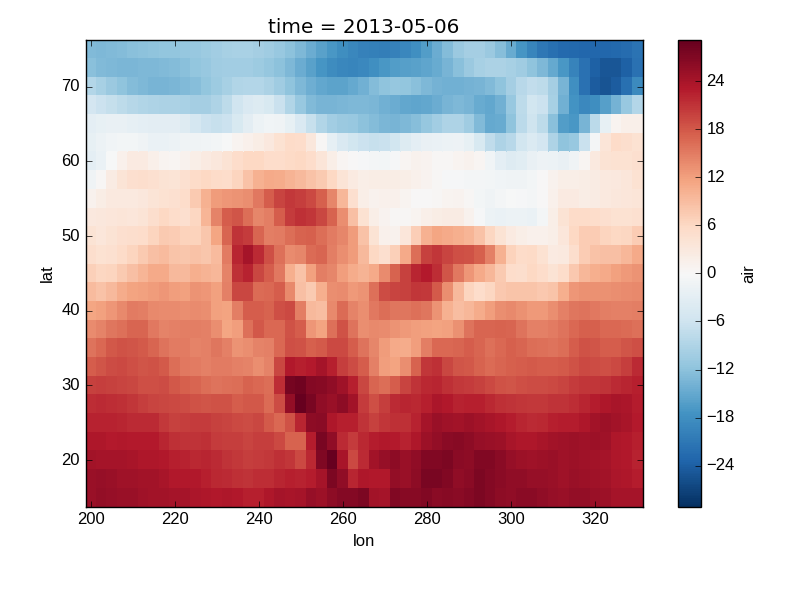All 2d plots in xarray allow the use of the keyword arguments yincrease and xincrease.

In : air2d.plot(yincrease=False)
Out: <matplotlib.collections.QuadMesh at 0x7fb79d695c10>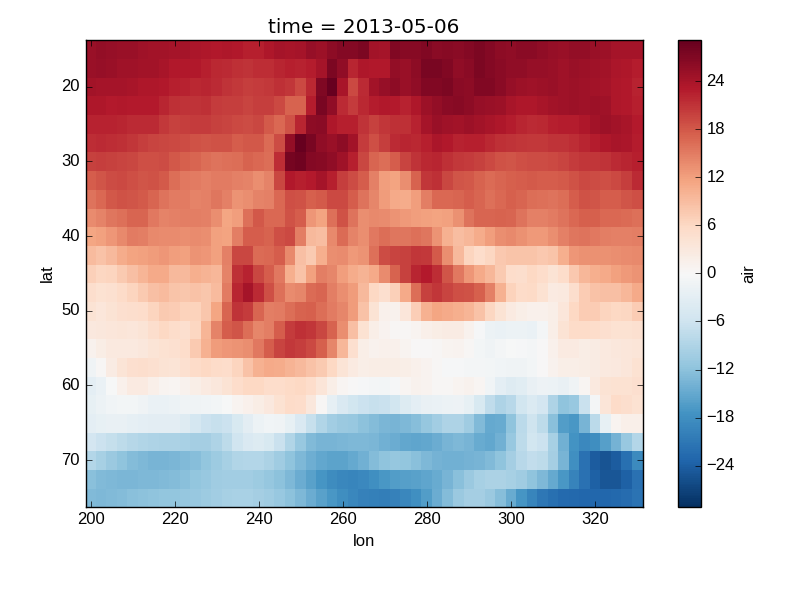Note

We use xarray.plot.pcolormesh() as the default two-dimensional plot method because it is more flexible than xarray.plot.imshow(). However, for large arrays, imshow can be much faster than pcolormesh. If speed is important to you and you are plotting a regular mesh, consider using imshow.

Missing Values¶

xarray plots data with Missing values.

In : bad_air2d = air2d.copy()

In : bad_air2d[dict(lat=slice(0, 10), lon=slice(0, 25))] = np.nan

Out: <matplotlib.collections.QuadMesh at 0x7fb794744610>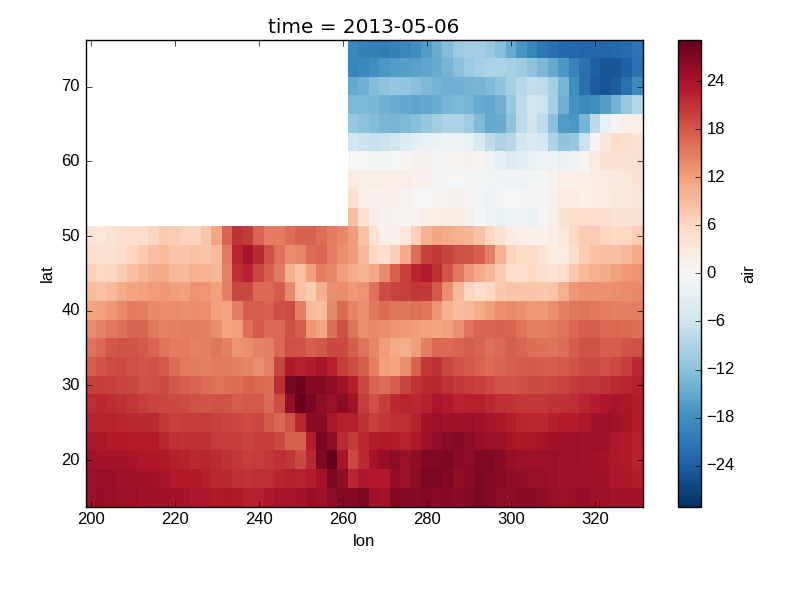Nonuniform Coordinates¶

It’s not necessary for the coordinates to be evenly spaced. Both xarray.plot.pcolormesh() (default) and xarray.plot.contourf() can produce plots with nonuniform coordinates.

In : b = air2d.copy()

# Apply a nonlinear transformation to one of the coords
In : b.coords['lat'] = np.log(b.coords['lat'])

In : b.plot()
Out: <matplotlib.collections.QuadMesh at 0x7fb7946abf10>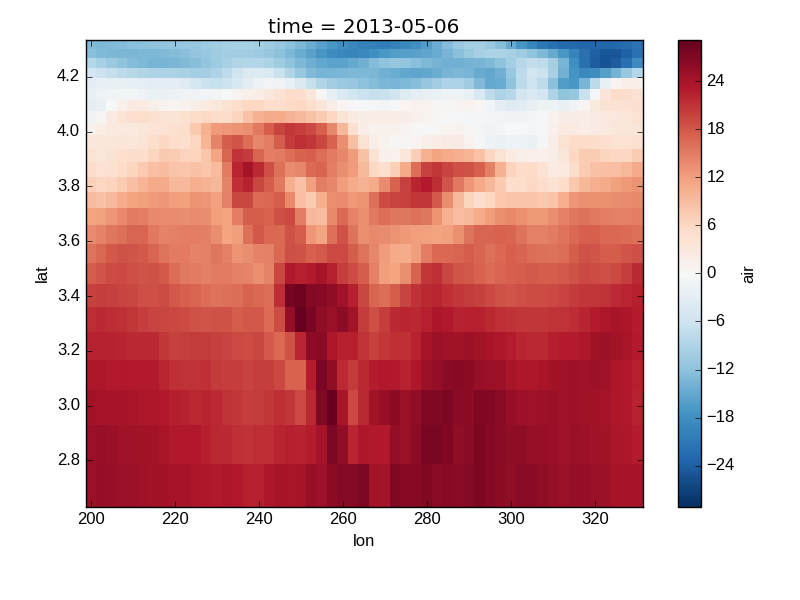Calling Matplotlib¶

Since this is a thin wrapper around matplotlib, all the functionality of matplotlib is available.

In : air2d.plot(cmap=plt.cm.Blues)
Out: <matplotlib.collections.QuadMesh at 0x7fb79d454610>

In : plt.title('These colors prove North America\nhas fallen in the ocean')
Out: <matplotlib.text.Text at 0x7fb794538650>

In : plt.ylabel('latitude')
Out: <matplotlib.text.Text at 0x7fb79457cd90>

In : plt.xlabel('longitude')
Out: <matplotlib.text.Text at 0x7fb7945e71d0>

In : plt.tight_layout()

In : plt.show()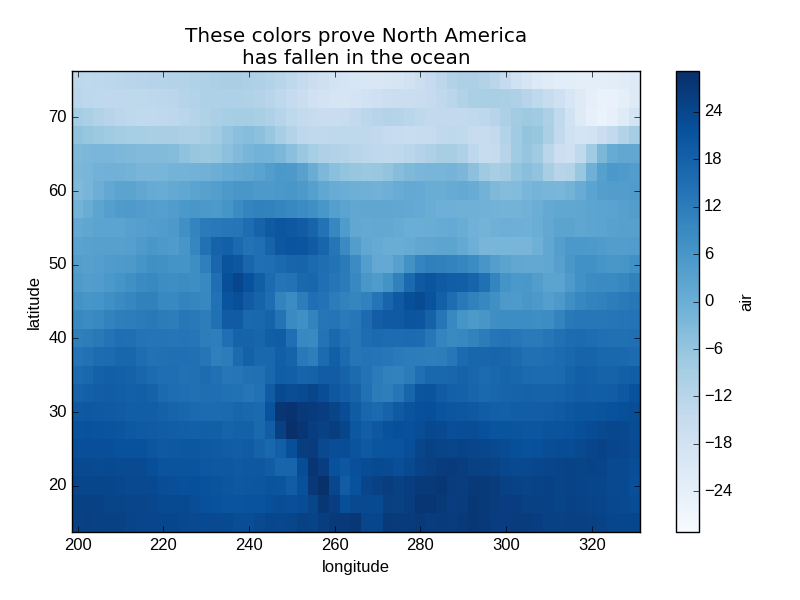Note

xarray methods update label information and generally play around with the axes. So any kind of updates to the plot should be done after the call to the xarray’s plot. In the example below, plt.xlabel effectively does nothing, since d_ylog.plot() updates the xlabel.

In : plt.xlabel('Never gonna see this.')
Out: <matplotlib.text.Text at 0x7fb7946f78d0>

In : air2d.plot()
Out: <matplotlib.collections.QuadMesh at 0x7fb794700710>

In : plt.show()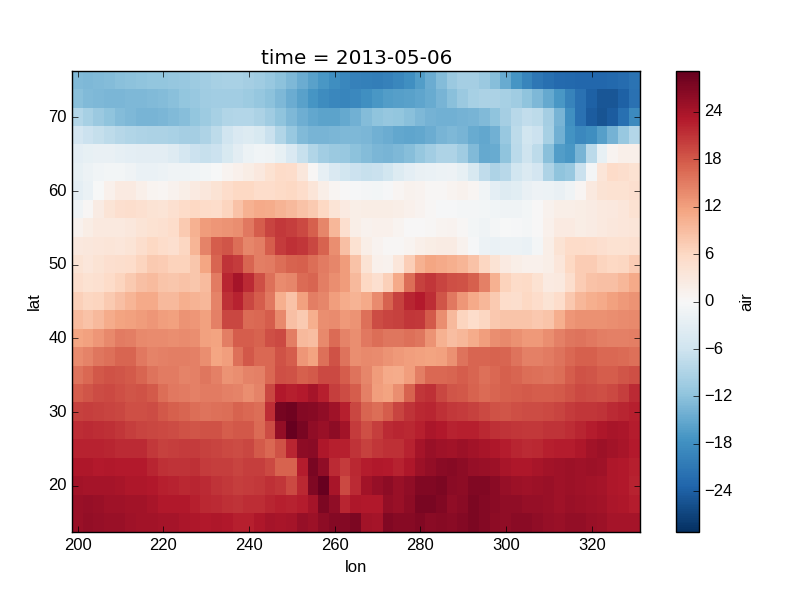Colormaps¶

xarray borrows logic from Seaborn to infer what kind of color map to use. For example, consider the original data in Kelvins rather than Celsius:

In : airtemps.air.isel(time=0).plot()
Out: <matplotlib.collections.QuadMesh at 0x7fb7944a9510>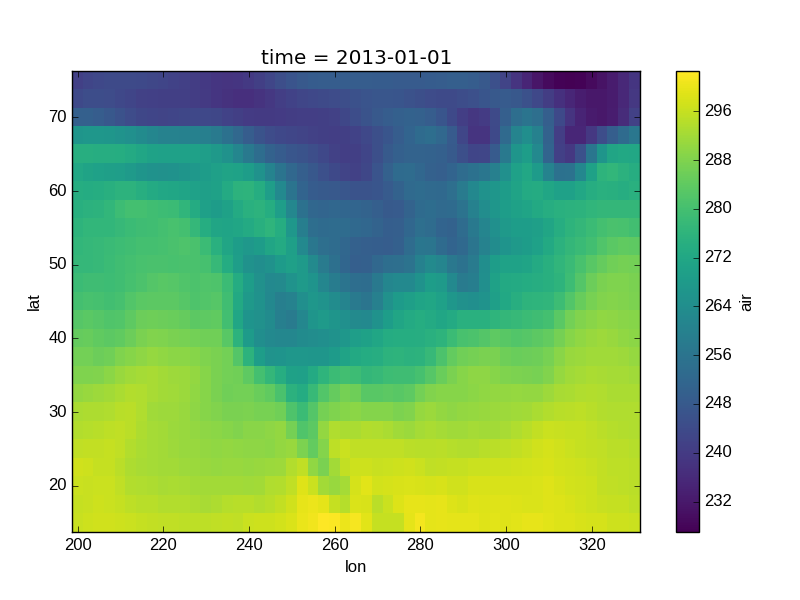The Celsius data contain 0, so a diverging color map was used. The Kelvins do not have 0, so the default color map was used.

Robust¶

Outliers often have an extreme effect on the output of the plot. Here we add two bad data points. This affects the color scale, washing out the plot.

In : air_outliers = airtemps.air.isel(time=0).copy()

In : air_outliers[0, 0] = 100

In : air_outliers[-1, -1] = 400

In : air_outliers.plot()
Out: <matplotlib.collections.QuadMesh at 0x7fb7943dbd10>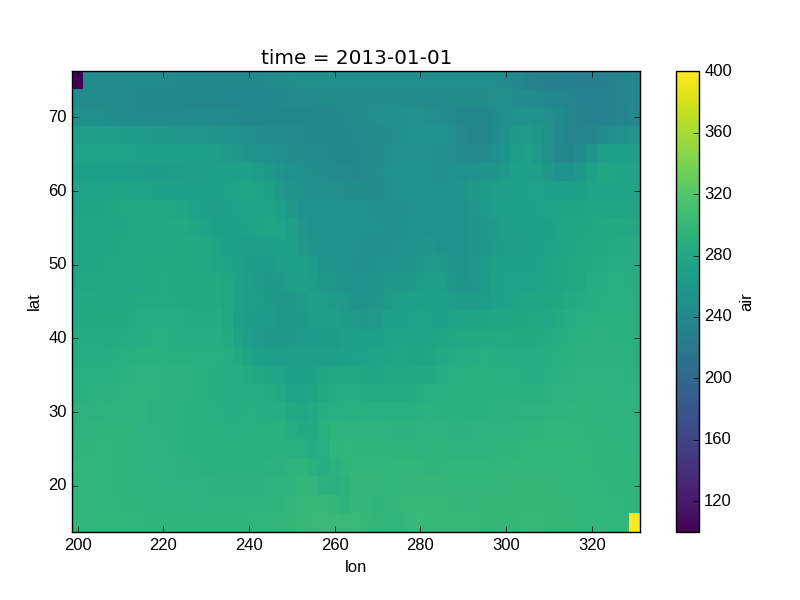This plot shows that we have outliers. The easy way to visualize the data without the outliers is to pass the parameter robust=True. This will use the 2nd and 98th percentiles of the data to compute the color limits.

In : air_outliers.plot(robust=True)
Out: <matplotlib.collections.QuadMesh at 0x7fb794257c10>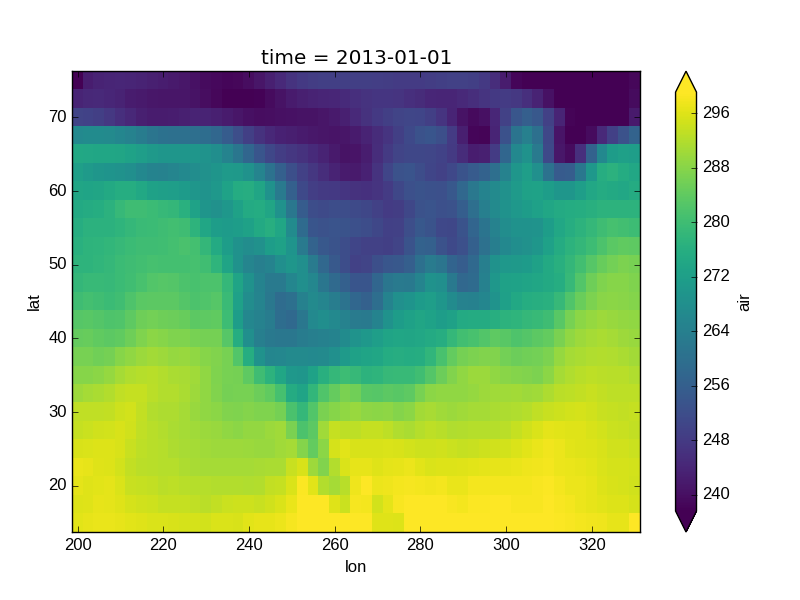Observe that the ranges of the color bar have changed. The arrows on the color bar indicate that the colors include data points outside the bounds.

Discrete Colormaps¶

It is often useful, when visualizing 2d data, to use a discrete colormap, rather than the default continuous colormaps that matplotlib uses. The levels keyword argument can be used to generate plots with discrete colormaps. For example, to make a plot with 8 discrete color intervals:

In : air2d.plot(levels=8)
Out: <matplotlib.collections.QuadMesh at 0x7fb79416a9d0>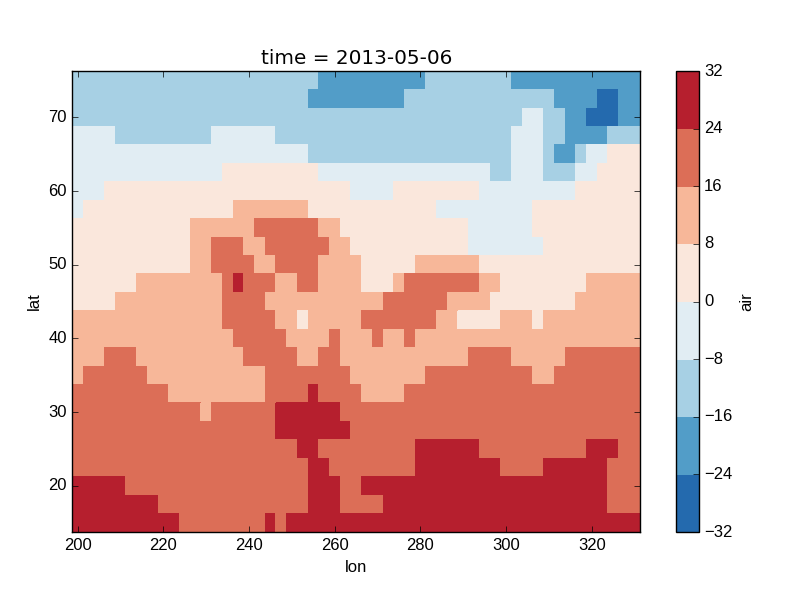It is also possible to use a list of levels to specify the boundaries of the discrete colormap:

In : air2d.plot(levels=[0, 12, 18, 30])
Out: <matplotlib.collections.QuadMesh at 0x7fb7942fb190>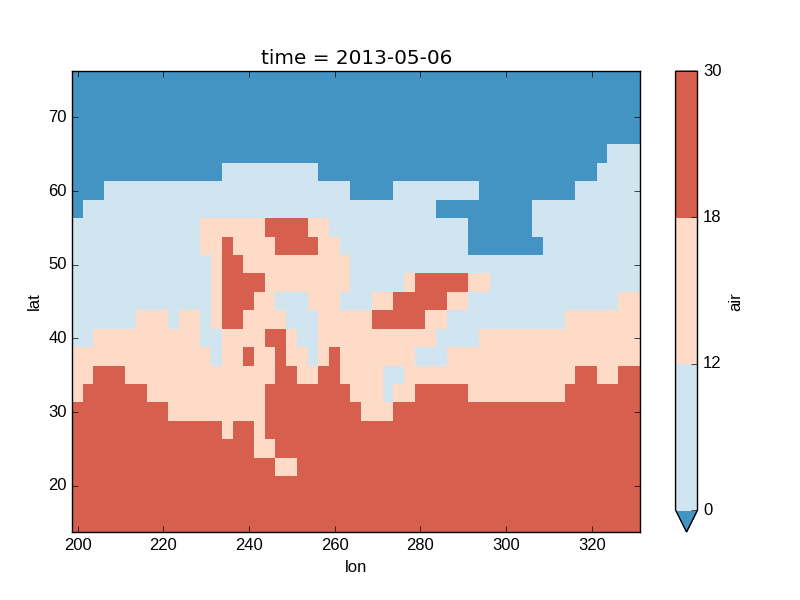You can also specify a list of discrete colors through the colors argument:

In : flatui = ["#9b59b6", "#3498db", "#95a5a6", "#e74c3c", "#34495e", "#2ecc71"]

In : air2d.plot(levels=[0, 12, 18, 30], colors=flatui)
Out: <matplotlib.collections.QuadMesh at 0x7fb7956492d0>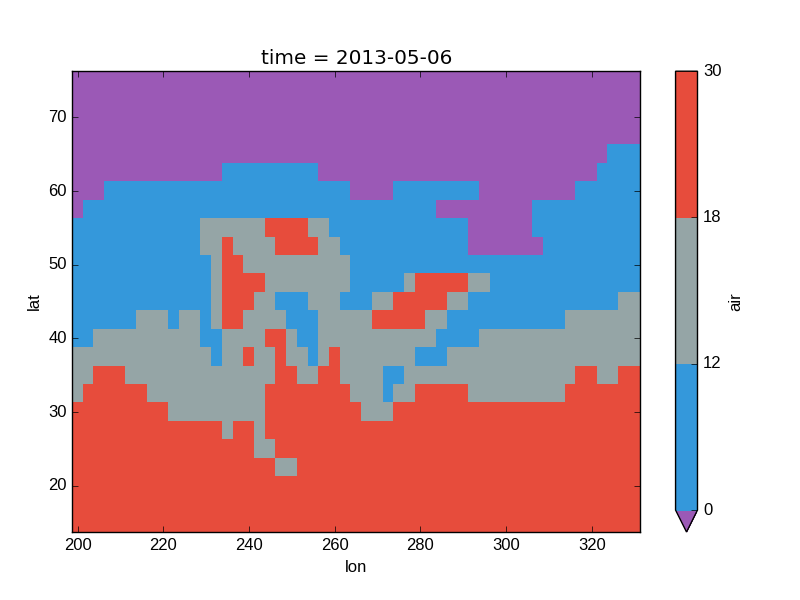Finally, if you have Seaborn installed, you can also specify a seaborn color palette to the cmap argument. Note that levels must be specified with seaborn color palettes if using imshow or pcolormesh (but not with contour or contourf, since levels are chosen automatically).

In : air2d.plot(levels=10, cmap='husl')
Out: <matplotlib.collections.QuadMesh at 0x7fb7903b3310>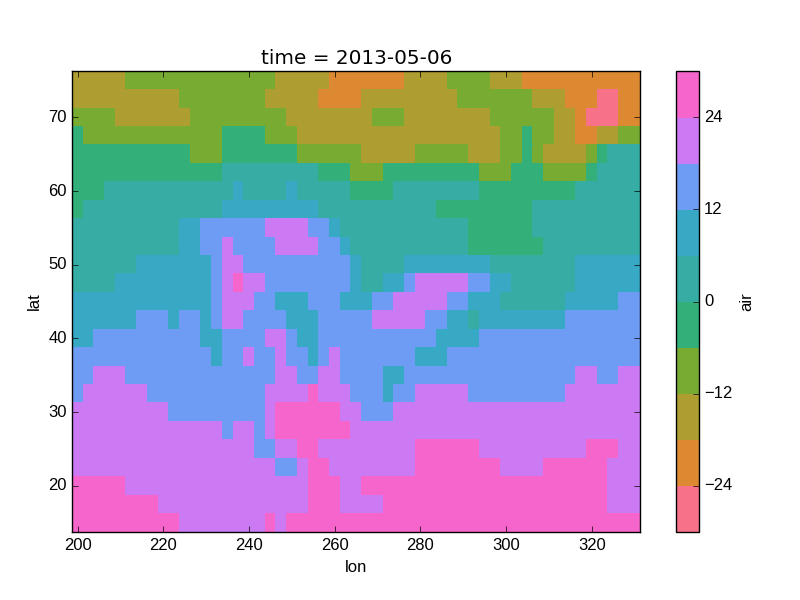Faceting¶

Faceting here refers to splitting an array along one or two dimensions and plotting each group. xarray’s basic plotting is useful for plotting two dimensional arrays. What about three or four dimensional arrays? That’s where facets become helpful.

Consider the temperature data set. There are 4 observations per day for two years which makes for 2920 values along the time dimension. One way to visualize this data is to make a seperate plot for each time period.

The faceted dimension should not have too many values; faceting on the time dimension will produce 2920 plots. That’s too much to be helpful. To handle this situation try performing an operation that reduces the size of the data in some way. For example, we could compute the average air temperature for each month and reduce the size of this dimension from 2920 -> 12. A simpler way is to just take a slice on that dimension. So let’s use a slice to pick 6 times throughout the first year.

In : t = air.isel(time=slice(0, 365 * 4, 250))

In : t.coords
Out:
Coordinates:
* lat      (lat) float32 75.0 72.5 70.0 67.5 65.0 62.5 60.0 57.5 55.0 52.5 ...
* time     (time) datetime64[ns] 2013-01-01 2013-03-04T12:00:00 2013-05-06 ...
* lon      (lon) float32 200.0 202.5 205.0 207.5 210.0 212.5 215.0 217.5 ...

Simple Example¶

The easiest way to create faceted plots is to pass in row or col arguments to the xarray plotting methods/functions. This returns a xarray.plot.FacetGrid object.

In : g_simple = t.plot(x='lon', y='lat', col='time', col_wrap=3)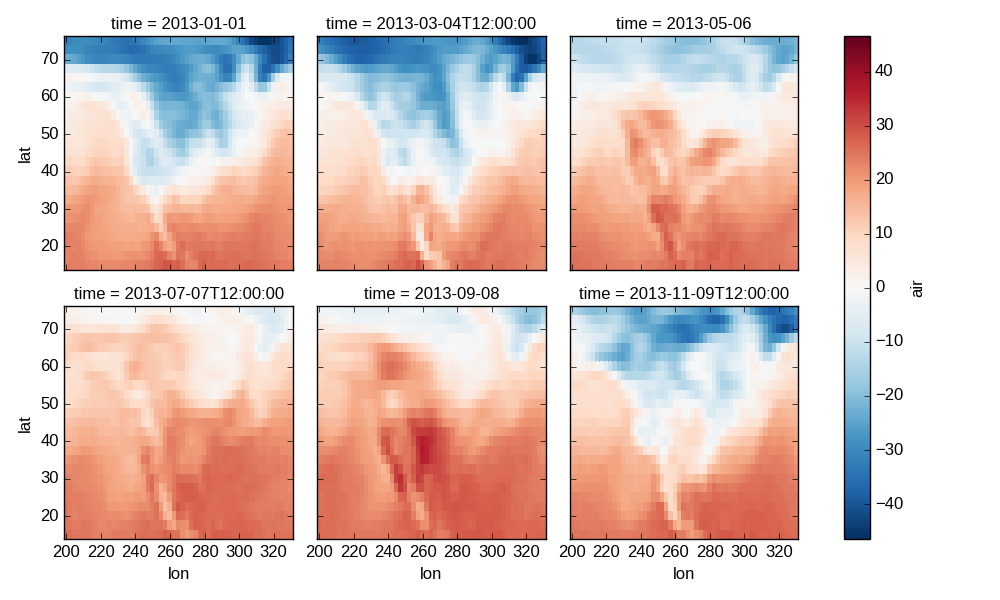4 dimensional¶

For 4 dimensional arrays we can use the rows and columns of the grids. Here we create a 4 dimensional array by taking the original data and adding a fixed amount. Now we can see how the temperature maps would compare if one were much hotter.

In : t2 = t.isel(time=slice(0, 2))

In : t4d = xr.concat([t2, t2 + 40], pd.Index(['normal', 'hot'], name='fourth_dim'))

# This is a 4d array
In : t4d.coords
Out:
Coordinates:
* lat         (lat) float32 75.0 72.5 70.0 67.5 65.0 62.5 60.0 57.5 55.0 ...
* time        (time) datetime64[ns] 2013-01-01 2013-03-04T12:00:00
* lon         (lon) float32 200.0 202.5 205.0 207.5 210.0 212.5 215.0 ...
* fourth_dim  (fourth_dim) object 'normal' 'hot'

In : t4d.plot(x='lon', y='lat', col='time', row='fourth_dim')
Out: <xarray.plot.facetgrid.FacetGrid at 0x7fb78fece1d0>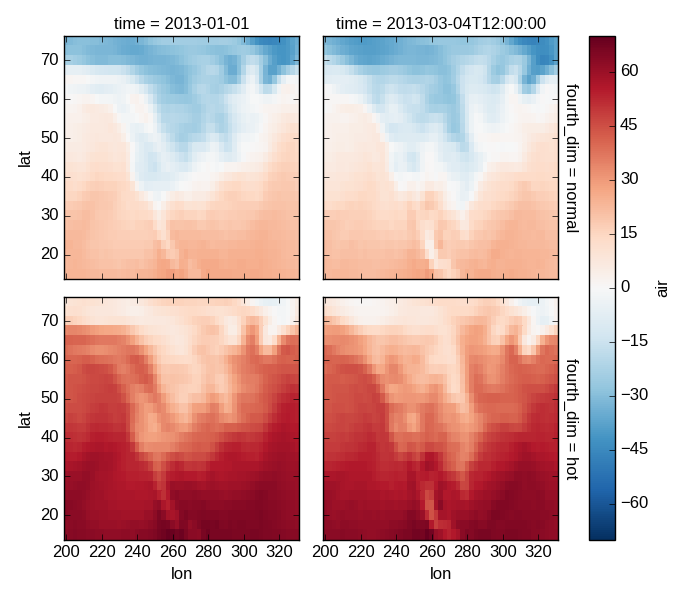Other features¶

Faceted plotting supports other arguments common to xarray 2d plots.

In : hasoutliers = t.isel(time=slice(0, 5)).copy()

In : hasoutliers[0, 0, 0] = -100

In : hasoutliers[-1, -1, -1] = 400

In : g = hasoutliers.plot.pcolormesh('lon', 'lat', col='time', col_wrap=3,
....:                                 robust=True, cmap='viridis')
....: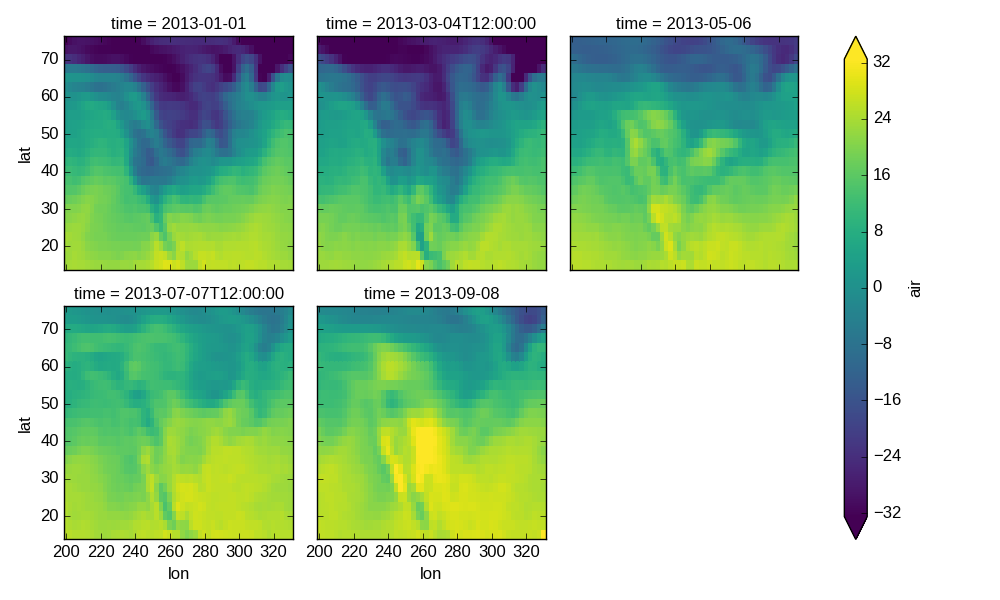FacetGrid Objects¶

xarray.plot.FacetGrid is used to control the behavior of the multiple plots. It borrows an API and code from Seaborn. The structure is contained within the axes and name_dicts attributes, both 2d Numpy object arrays.

In : g.axes
Out:
array([[<matplotlib.axes._subplots.AxesSubplot object at 0x7fb78fc73f90>,
<matplotlib.axes._subplots.AxesSubplot object at 0x7fb78fbcc790>,
<matplotlib.axes._subplots.AxesSubplot object at 0x7fb78fbad510>],
[<matplotlib.axes._subplots.AxesSubplot object at 0x7fb78fb2f710>,
<matplotlib.axes._subplots.AxesSubplot object at 0x7fb78fb47850>,
<matplotlib.axes._subplots.AxesSubplot object at 0x7fb78fa14e10>]], dtype=object)

In : g.name_dicts
Out:
array([[{'time': numpy.datetime64('2013-01-01T00:00:00.000000000+0000')},
{'time': numpy.datetime64('2013-03-04T12:00:00.000000000+0000')},
{'time': numpy.datetime64('2013-05-06T00:00:00.000000000+0000')}],
[{'time': numpy.datetime64('2013-07-07T12:00:00.000000000+0000')},
{'time': numpy.datetime64('2013-09-08T00:00:00.000000000+0000')}, None]], dtype=object)

It’s possible to select the xarray.DataArray or xarray.Dataset corresponding to the FacetGrid through the name_dicts.

In : g.data.loc[g.name_dicts[0, 0]]
Out:
<xarray.DataArray 'air' (lat: 25, lon: 53)>
array([[-100.  ,  -30.65,  -29.65, ...,  -40.35,  -37.65,  -34.55],
[ -29.35,  -28.65,  -28.45, ...,  -40.35,  -37.85,  -33.85],
[ -23.15,  -23.35,  -24.26, ...,  -39.95,  -36.76,  -31.45],
...,
[  23.45,   23.05,   23.25, ...,   22.25,   21.95,   21.55],
[  22.75,   23.05,   23.64, ...,   22.75,   22.75,   22.05],
[  23.14,   23.64,   23.95, ...,   23.75,   23.64,   23.45]])
Coordinates:
* lat      (lat) float32 75.0 72.5 70.0 67.5 65.0 62.5 60.0 57.5 55.0 52.5 ...
time     datetime64[ns] 2013-01-01
* lon      (lon) float32 200.0 202.5 205.0 207.5 210.0 212.5 215.0 217.5 ...

Here is an example of using the lower level API and then modifying the axes after they have been plotted.

In : g = t.plot.imshow('lon', 'lat', col='time', col_wrap=3, robust=True)

In : for i, ax in enumerate(g.axes.flat):
....:     ax.set_title('Air Temperature %d' % i)
....:

In : bottomright = g.axes[-1, -1]

In : bottomright.annotate('bottom right', (240, 40))
Out: <matplotlib.text.Annotation at 0x7fb78f82dc10>

In : plt.show()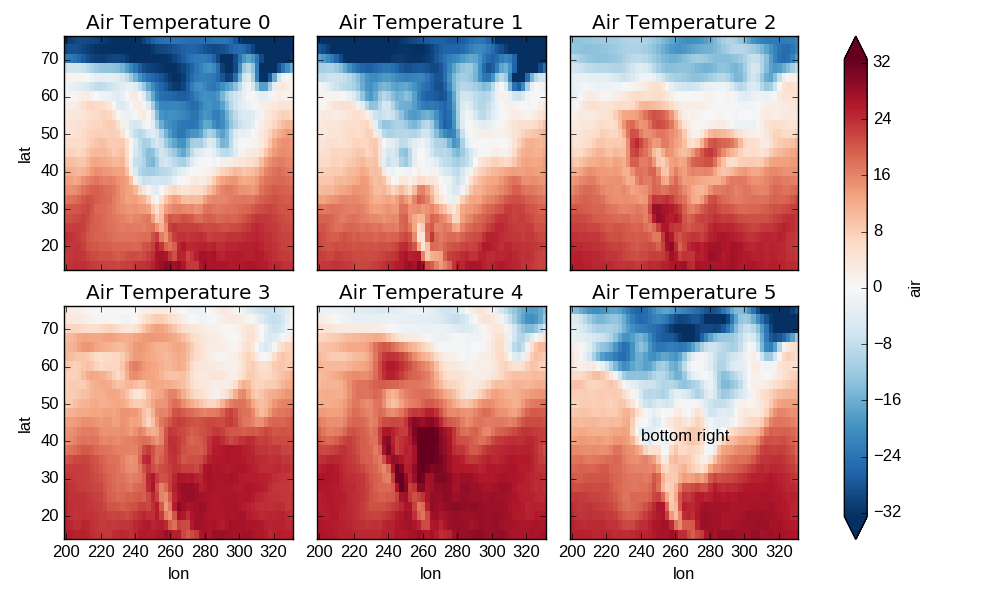TODO: add an example of using the map method to plot dataset variables (e.g., with plt.quiver).

Maps¶

To follow this section you’ll need to have Cartopy installed and working.

This script will plot the air temperature on a map.

import xarray as xr
import matplotlib.pyplot as plt
import cartopy.crs as ccrs

air = (xr.tutorial
.air
.isel(time=0))

ax = plt.axes(projection=ccrs.Orthographic(-80, 35))
ax.set_global()
air.plot.contourf(ax=ax, transform=ccrs.PlateCarree())
ax.coastlines()

plt.savefig('cartopy_example.png')

Here is the resulting image: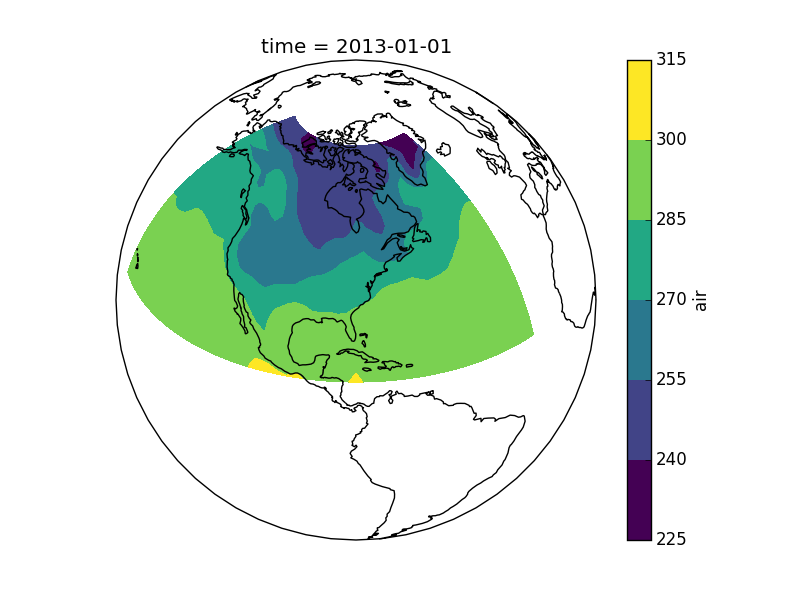Details¶

Ways to Use¶

There are three ways to use the xarray plotting functionality:

1. Use plot as a convenience method for a DataArray.
2. Access a specific plotting method from the plot attribute of a DataArray.
3. Directly from the xarray plot submodule.

These are provided for user convenience; they all call the same code.

In : import xarray.plot as xplt

In : da = xr.DataArray(range(5))

In : fig, axes = plt.subplots(ncols=2, nrows=2)

In : da.plot(ax=axes[0, 0])
Out: [<matplotlib.lines.Line2D at 0x7fb78f358ed0>]

In : da.plot.line(ax=axes[0, 1])
Out: [<matplotlib.lines.Line2D at 0x7fb79d643650>]

In : xplt.plot(da, ax=axes[1, 0])
Out: [<matplotlib.lines.Line2D at 0x7fb78f54d910>]

In : xplt.line(da, ax=axes[1, 1])
Out: [<matplotlib.lines.Line2D at 0x7fb78f54d950>]

In : plt.tight_layout()

In : plt.show()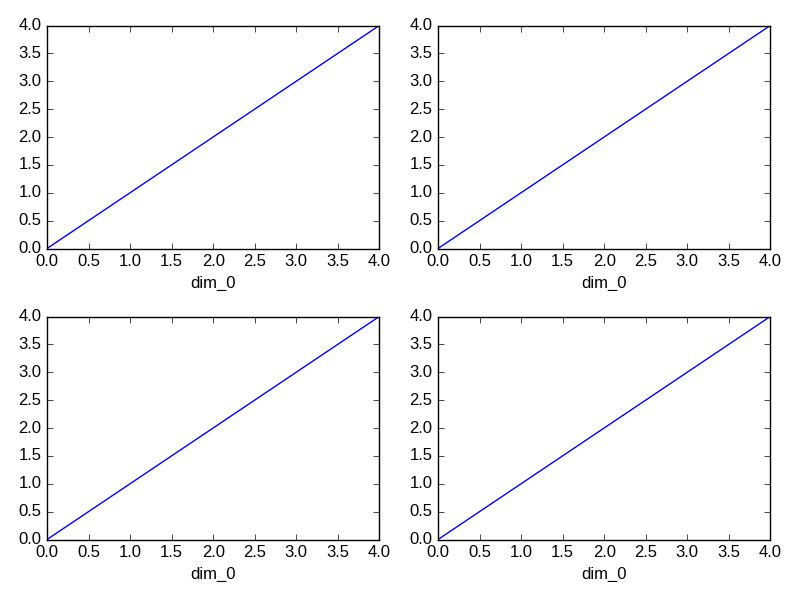Here the output is the same. Since the data is 1 dimensional the line plot was used.

The convenience method xarray.DataArray.plot() dispatches to an appropriate plotting function based on the dimensions of the DataArray and whether the coordinates are sorted and uniformly spaced. This table describes what gets plotted:

 Dimensions Plotting function 1 xarray.plot.line() 2 xarray.plot.pcolormesh() Anything else xarray.plot.hist()

Coordinates¶

If you’d like to find out what’s really going on in the coordinate system, read on.

In : a0 = xr.DataArray(np.zeros((4, 3, 2)), dims=('y', 'x', 'z'),
....:                   name='temperature')
....:

In : a0[0, 0, 0] = 1

In : a = a0.isel(z=0)

In : a
Out:
<xarray.DataArray 'temperature' (y: 4, x: 3)>
array([[ 1.,  0.,  0.],
[ 0.,  0.,  0.],
[ 0.,  0.,  0.],
[ 0.,  0.,  0.]])
Coordinates:
* y        (y) int64 0 1 2 3
* x        (x) int64 0 1 2
z        int64 0

The plot will produce an image corresponding to the values of the array. Hence the top left pixel will be a different color than the others. Before reading on, you may want to look at the coordinates and think carefully about what the limits, labels, and orientation for each of the axes should be.

In : a.plot()
Out: <matplotlib.collections.QuadMesh at 0x7fb78f257d10>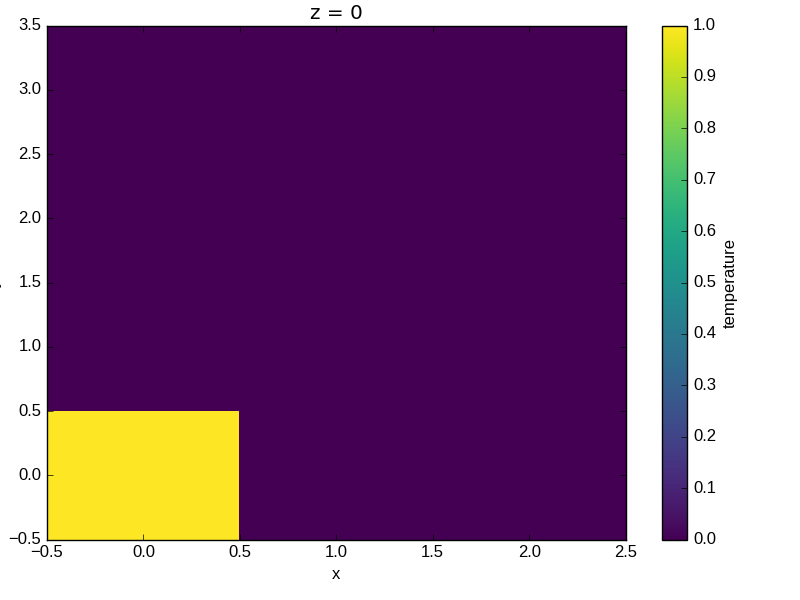It may seem strange that the values on the y axis are decreasing with -0.5 on the top. This is because the pixels are centered over their coordinates, and the axis labels and ranges correspond to the values of the coordinates.